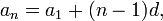## Sunday, March 28, 2010

### Cubase 5: Turning inactive to activeIf you accidentally disconnected an audio device in the C5's Device Setup>VST Audio System>ASIO, hit F4, or go to Device>VST Connection, to open the VST Connection panel, change the both Stereo's left and right bus to the name of the disconnected device. The disconnected audio will then be connected again.

## Friday, March 26, 2010

### Array.shuffle()

Array.shuffle(), in reality doesn't exist in Flash's AS3, thus it's a pain in the ass for us, fellow moderate-level AS3 programmer.

His script here,
``var arr2:Array = [];while (arr.length > 0) {    arr2.push(arr.splice(Math.round(Math.random() * (arr.length - 1)), 1));}``
"Tells" the AS3 to execute a function which shuffle an Array of stuff into another new Array.

## Wednesday, March 24, 2010

### Revisiting Arithmetic progression

I was making a typing game for my class's assignment using Unity3D and Java, and I'm trying to create a level script for my game. There's a few ways I could do it if I look for it on the internet on in the Unity forum, but for this time, I wanna try scripting it myself.

I try on a few ways I could think of (like using the "if, else" statement, and create a really long script about when the level should change when the scores hits a certain point -- which is really a time and space wasting effort), before I realized about Arithmetic progression, an additional-math formula I learnt in high school.

I first list down a few levels, and the scores required to reach them. for instance:
0 scores-level 1 (I wanted the player to start at level 1, with 0 scores),
10000 scores - level 2,
20000 scores - level 3,
30000 scores - level 4, etc...

The difference between 2 of those levels is 10000 scores. Using these infos, I started working it in the Arithmetic progression formula:(from Wikipedia, I've totally forgotten about the formula after quitting math since 2 years ago)

a (with a small n) = is the player scores here...
a (with a small 1) = is the starting scores, which is 0 (refer above)...
n (inside the bracket) = refer to the player's level...
d = the difference between 2 of those levels, which is 10000 (refer above)...

The structure of the formula in script would look like this:
``var scores:int = 0;var level:int = 0;var diff:int = 10000;var aOne:int = 0; //these are required variables set//the formulascores = 0 + (level - 1) * diff;``
Since I'm gonna change the value of level here instead of the scores, I need to change the formula a bit to:
``level = (scores - aOne) / diff + 1;``
Then, applying it to the main script:
``var scores:int = 0;var level:int = 0;var diff:int = 10000;var aOne:int = 0;function Update () {if ((scores - aOne) / diff + 1 > level) {level ++;}``
What the script is saying here is that, if the result of the calculation is more than player's level, the level will increase itself by the value of one.

Say... I've managed to score around 11300 points while I'm in level 1. Using the formula:
(11300 - 0) / 10000 + 1, the result would be 1.13, which is more than 1 (the current level)... Thus, the level will increase itself by 1, and become level 2...

If I were to create some sorts of a RPG/first-person-shooter game, a Geometric progression would be the better choice in writing the level script.